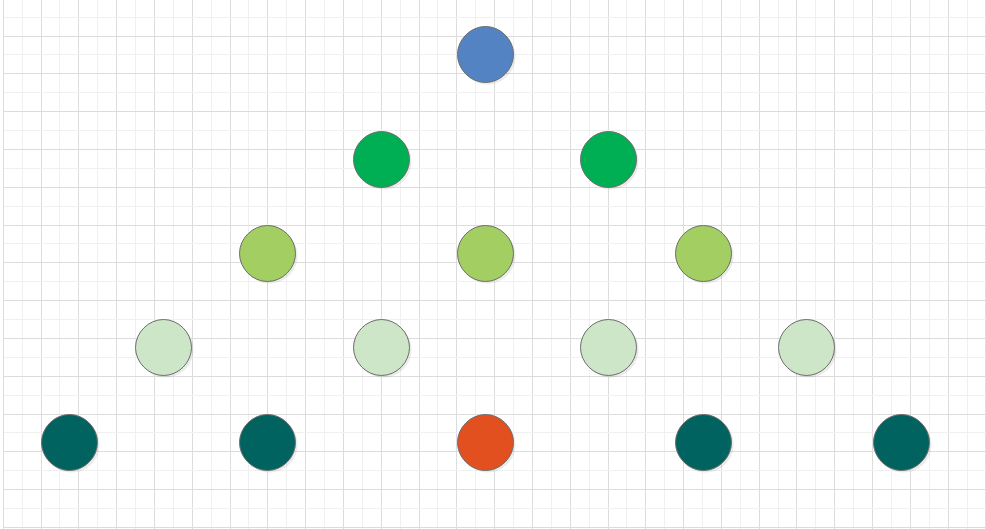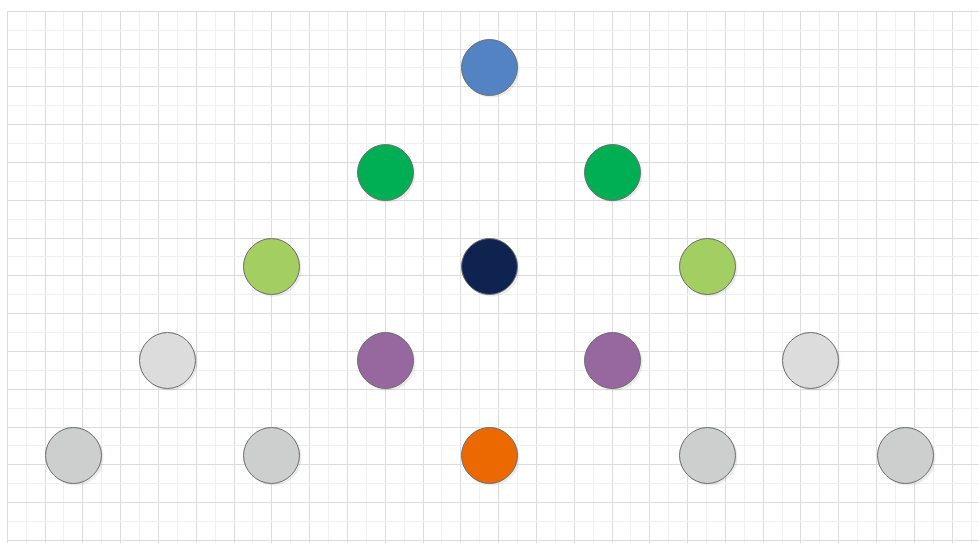• 简单BFS算法 C++实现（未注释） 21.10.1
2021-10-03 09:50:34

int main()
{
queue<int> search;
int group = {1,3,5,7,9,11,13,15,17};
for (int i = 0; i < 3; i++)
{
search.push(group[i]);
}

int research(queue<int> search,int group[]);

research(search, group);

}
int research(queue<int> search, int group)
{

//cout << "测试点1 people=" << people;
int i = 0,j=1;
while (search.size())
{
int people = search.front();
//cout << "测试点2 people=" << people;

if (people == 11)
{
cout << "找到了" << people;
return 1;

}

else
{
//cout << "测试点3 people=" << people;
if (i == 3)
{
j++;
i = 0;
}
search.pop();
search.push(group[j][i]);
i++;

}

}
return 0;
}

更多相关内容
• } } BFS void BFS(AGraph *G,int v,int visit[maxSize]) { ArcNode *p; int que[maxSize],front=0,rear=0; int j; printf("%c\n",G->adjlist[v].data); visit[v]=1; rear=(rear+1)%maxSize; que[rear]=v; ...

结构体
typedef struct ArcNode
{
struct ArcNode *nextarc; /指向下一条边/
int info; //可以表示权值
}ArcNode;

typedef struct
{
char data; /顶点域/
ArcNode *firstarc; /表头指针/
}VNode;

typedef struct AGraph
{
int n,e; /顶点数和边数/
}AGraph;

创建图

AGraph* Create_Graph( )
{

AGraph *G;
G=(AGraph *)malloc(sizeof(AGraph));   //分配内存很重要，不然错在哪都不知道
int i;
printf("请输入顶点数和边数(输入格式为:顶点数,边数)\n");
//	cin>>G->n>>G->e;
scanf("%d %d",&(G->n),&(G->e)); /*读入顶点数和边数*/
printf("请输入顶点信息:\n");
for (i=0;i<G->n;i++) /*建立有n 个顶点的顶点表*/
{
}
printf("请输入边的信息(输入格式为:i,j)：\n");
//	printf("请输入边的信息(输入格式为:i,j,info)：\n");
int j,k;
ArcNode *s;
for (k=0;k<G->e;k++)     /*建立边表*/
{
cin>>i>>j;
//	scanf("%d,%d\n",&i,&j,&m);   /*读入边<Vi,Vj>的顶点对应序号*/
s=(ArcNode*)malloc(sizeof(ArcNode)); /*生成新边表结点s*/
//	s->info=m;

/*无向图再加上这个
s=(ArcNode*)malloc(sizeof(ArcNode));
//		s->info=m;
}
cout << "邻接表如下：" << endl;
for (int i = 0; i < G->n; i++) {
while (p!=NULL)
{
p = p->nextarc;
}
cout<<endl;
}
return G;
}


DFS

int visit[maxSize]={0};
void DFS(AGraph *G,int v)
{
ArcNode *p;
visit[v]=1;
while(p!=NULL)
{
p=p->nextarc;
}
}



BFS

void BFS(AGraph *G,int v,int visit[maxSize])
{
ArcNode *p;
int que[maxSize],front=0,rear=0;
int j;
visit[v]=1;
rear=(rear+1)%maxSize;
que[rear]=v;
while(rear!=front)
{
front=(front+1)%maxSize;
j=que[front];
while(p!=NULL)
{
{
rear=(rear+1)%maxSize;
}
p=p->nextarc;
}

}
}



示例

int main()
{
AGraph *G;
G=Create_Graph();
//	DFS(G,0);
BFS(G,0,visit);
}
/*
请输入顶点信息:

请输入边的信息(输入格式为:i j)：
5 7
0
1
2
3
4
0 1
0 3
0 4
1 2
1 4
2 0
3 2

*/

展开全文dfs
• } } } void BFS(int v) { visit(v); visited[v] = true; queue<int> Q; Q.push(v); while (!Q.empty()) { int f = Q.front(); Q.pop(); for (int w = 0; w ; w++) { if (edge[w][f] == 1 && visited[w] == false) {...

使用邻接矩阵存储图

#include <iostream>
#include <queue>
using namespace std;

int vertex; // 顶点数组
int edge; // 边数组
bool visited; // 访问标记
int n; // 顶点个数

void visit(int v) {
cout << vertex[v] << "  ";
}

void DFS(int v) {
visit(v); // 访问顶点元素
visited[v] = true;
for (int w = 0; w < n; w++) {
if (edge[v][w] == 1 && visited[w] == false) {
visited[w] = true;
DFS(w);
}
}
}

void BFS(int v) {
visit(v);
visited[v] = true;
queue<int> Q;
Q.push(v);
while (!Q.empty()) {
int f = Q.front();
Q.pop();
for (int w = 0; w < n; w++) {
if (edge[w][f] == 1 && visited[w] == false) {
visit(w);
visited[w] = true;
Q.push(w);
}
}
}
}

int main() {
n = 8; // 顶点个数

/*--------------初始化-----------------*/
for (int i = 0; i < n; i++) {
vertex[i] = i;
}
for(int i = 0; i < n; i++) {
for (int j = 0; j < n; j++) {
if (i == j)
edge[i][j] = 0;
else edge[i][j] = 0x3fffffff;
}
}
edge = 1; edge = 1;
edge = 1; edge = 1;
edge = 1; edge = 1;
edge = 1; edge = 1;
edge = 1; edge = 1;
edge = 1; edge = 1;
edge = 1; edge = 1;
edge = 1; edge = 1;
edge = 1; edge = 1;
for (int i = 0; i < n; i++) {
visited[i] = false;
}
/*--------------初始化-----------------*/

cout << "==============下面是DFS==================" << endl;
for (int i = 0; i < n; i++) {
if (!visited[i])
DFS(i);
}
cout << endl;
cout << "==============下面是BFS==================" << endl;
for (int i = 0; i < n; i++) {
visited[i] = false;
}
for (int i = 0; i < n; i++) {
if (!visited[i])
BFS(i);
}
cout << endl;
return 0;
}

展开全文• DFS和BFS算法的实现，使用C++语言，适合数据结构初学者学习。
• ## 【C++】算法集锦（5）：BFS算法

千次阅读 多人点赞 2021-02-21 11:36:35
BFS算法框架 BFS算法和DFS算法属于图论算法的范畴，DFS在前面回溯中，可以去看一下。 BFS算法用于寻找两点之间的最短路径。 碧如说：寻找树的最小高度（迭代法）、走迷宫、导航等问题。 这些问题看起来都会比较抽象...## BFS算法框架

BFS算法和DFS算法属于图论算法的范畴，DFS在前面回溯中，可以去看一下。
BFS算法用于寻找两点之间的最短路径。

碧如说：寻找树的最小高度（迭代法）、走迷宫、导航等问题。
这些问题看起来都会比较抽象，去做也是很抽象。

与其说算法框架难写，倒不如说是把实际问题转化为算法问题来的要难。
还记得我在图论算法那篇里面有讲过：学习图论算法，最难的是要有用图论算法的意识。等下看了例题就知道了。

### 框架代码

这个代码其实就是微调一下图的BFS遍历，搞成个伪代码的样子，没什么新鲜的。

int BFS(Node start,Node target){
/*
这是一个BFS算法的代码框架
return：返回从start到target的最短步数
start：起始点
target：终点
*/

Queue<Node> q;
Set<Node> visited;	//避免走回头路

q.offer(start);	//将起点加入队列
int step = 0;	//纪录扩散的步数

while(q not empty){
int sz = q.size();
for(int i = 0; i<sz; i++){
Node cur = q.poll();

//判断是否到终点
if(cur is target)
return step;

//将cur相邻节点加入队列
if(x not in visited){
q.offer(x);
}
}
step++;	//更新步数
}
}


## 简单题：二叉树的最小高度

不难吧，用递归的话一下就出来了。
不过现在不是在讲BFS嘛，那就用BFS的方法吧。

起点是什么？起点是根节点。终点是什么？终点就是最靠近根节点的、两个子节点都是Null的节点。

接下来，我们对上面的框架进行改造：

int minDepth(TreeNode root){
/*
这是一个求二叉树最小高度的函数
return：二叉树的最小高度
root：根节点
*/
if(root == NULL) return 0;

Queue<TreeNode> q;

q.offer(root);	//将起点加入队列
int depth = 1;

while(!q.isEmpty()){
int sz = q.size();
for(int i = 0; i<sz; i++){
TreeNode cur = q.poll();

//判断是否到终点
if(cur.left == NULL && cur.right == NULL)
return depth;

//将cur相邻节点加入队列
if(cur.left != NULL)
q.offer(cur.left);
if(cur.right != NULL)
q.offer(cur.right);
}
depth++;	//更新步数
}
return depth;
}


## 拔高题：解开密码锁的最少次数

你有一个带有四个圆盘拨轮的轮盘锁，每个拨轮都有“0-9”十个数字，旋转没有边界限制，但是每次只能旋转一个位置。轮盘锁的初始位置是“0000”，现在给你一个密码和一组死亡密码（避免拨出的密码），请你设计一个算法，计算从初始状态到拨出最终密码所需要的最少次数。

抽象吧，就直接看这个题目，直接给我干懵逼了。
但是，不怕啊，前面不是说过了动态规划类题目的解题步骤嘛，先把暴力解法画出来，走通一条路，再优化。

那，暴力解法怎么解？真的，要不是有那个“死亡密码组”的存在，还真的就很暴力了。

第一步，拨一下。不管会怎么样，都得拨一下吧。这一下有八种可能了吧。
第二步，匹配。拨一下，对所有结果都进行一次的匹配。如果对上了，就返回结果；如果对不上，返回第一步再循环。
注意点一：如果碰到死亡密码，跳过。
注意点二：不要走回头路。
注意点三：空间能省着用就省着用。


好，关键的一步来了，怎么将这个暴力算法往图论算法的方向去引呢。
再看一下上面这个暴力算法，不难看出来，这就是一个节点下面拖八个子节点的八叉树，又是求最短距离，BFS。

int openLock(String[] deadends,String target){
//纪录需要跳过的死亡密码

//纪录已经穷举过的密码
set<String> visited = new HashSet<>();

//从起点开始启动广度优先搜索
int step = 0;
q.offer("0000");

while(!q.isEmpty){
int sz = q.size();
for(int i = 0;i<sz;i++){
string cur = q.poll();

//判断密码是否合法
continue;
if(cur.equals(target))
return step;
}

//将一个节点的未遍历相邻节点加入队列
for(int j=0;j<4;j++){
String up = plusOne(cur,j);
if(!visited.contains(up){
q.offer(up);
}
String down = minusOne(cur,j);
if(!visited.contains(down){
q.offer(down);
}
}
step++;
}
return -1;
}


上翻下翻的代码：

String plusOne(String s,int j){
char[] ch = s.toCharArray();
if(ch[j] == '9')
ch[j] = '0';
else
ch[j] += 1;
return new String(ch);
}

String downOne(String s,int j){
char[] ch = s.toCharArray();
if(ch[j] == '0')
ch[j] = '9';
else
ch[j] -= 1;
return new String(ch);
}


### 一波优化：双向BFS

上面的操作，简化一下是这样的：从顶部蓝色的节点，找底部红色的节点。

所有节点都被遍历了。

这时候我们换个思路，既然终点也是已知的，那就：上下同步进行，在中间黑色节点的地方汇聚了。

展开全文• 前辈创作不易，字字皆是...广度优先搜索（也称宽度优先搜索，缩写BFS，以下采用广度来描述）是连通图的一种遍历策略。因为它的思想是从一个顶点V0开始，辐射状地优先遍历其周围较广的区域，故得名。  一般可以用...
• ## C++算法——BFS（图解）

万次阅读 多人点赞 2019-09-02 16:45:46
宽度优先搜索算法（又称广度优先搜索）是最简便的图的搜索算法之一，这一算法也是很多重要的图的算法的原型。其别名又叫BFS，属于一种盲目搜寻法，目的是系统地展开并检查图中的所有节点，以找寻结果。换句话说，它...
• 深度优先搜索（Depth-First-Search）： 事实上，深度优先搜索属于... BFS并不使用经验法则算法。从算法的观点，所有因为展开节点而得到的子节点都会被加进一个先进先出的队列中。一般的实验里，其邻居节点尚未被检验
• 图的广度优先遍历算法 #define MaxSize 1000 // 边节点 typedef struct ANode { // 边所指的终点顶点 int adjvex; // 下一条边，邻域，指向下一个邻接点 struct ANode * nextarc; // 权值 int weight; }...数据结构
• 200. 岛屿数量(DFS算法 C++带详细注释） class Solution { public: // void dfs(vector<vector<char>>& grid,int i, int j)//DFS遍历函数 // { int row=grid.size();//矩阵行数 // int col=grid....dfs leetcode 队列
• bfs就是广度优先搜索，是最简便的图的搜索算法之一，这一算法也是很多重要的图的算法的原型。 ⒈从图中的某一顶点V0开始，先访问V0； ⒉访问所有与V0相邻接的顶点V1，V2，......，Vt； ⒊依次访问与V1，V2，.........宽度优先
• BFS算法： 类似于树的层序遍历，需要借助队列实现 算法的基本流程：起始点——探索未遍历的邻接点——未遍历的邻接点作为新的起始点重复 #include <iostream> #include <vector> #include <queue&...深度优先
• //BFS算法用到 int sum;//保存达到某一步时最大金币数， int maxT ;//最大的时间,即需寻找的dp最大行数 int a ;//左移，不变，右移 int curT ;//记录当前行索引，如果本行搜索完毕则更新数据 bool ...
• BFS我们称之为宽搜，通常可以用于解决最短，最小问题。不同于深搜，宽搜每次先把同一层的遍历一遍，若无正确答案再去遍历下一层，因此不需要用到递归，只需要用到循环即可。 先来看一道经典例题：走迷宫 解决走...
• 没啥事写的一个C++解最小步数二阶魔方的程序，cpp 200多行，尽力写的比较精简，大多是格式化设计（函数列表等），可扩展性比较强，估计改个2~30行代码就能改成3阶魔方。 用的是广搜（BFS），效率算是比较高，平均...
• C++基本算法讲解之广度优先搜索（BFS） 今天来讲算法了。 今天要用到基本队列，不知道的去看看我的上一次博文C++3.4数据结构之队列基础+blah数集题解 。 广度优先搜索（BFS）是从初始节点开始，根据搜索规则生成第...队列
• 2. BFS – use iteration(queue)   void bfs(TreeNode* root) {  if(root){  queue*> q;  q.push(root);    while(!q.empty()){  for(int i = 0, n = q.size(); i ; i++){  TreeNode* node ...
• ## C++DFS与BFS实现

千次阅读 2020-11-22 19:50:45
C++DFS与BFS实现DFSBFS   BFS使用一个queue实现，每次循环对当前点临近的点，都推进队列中，然后就能根据队列来依次访问临近的点，直到队列中没有点为止。   DFS利用一个stack，首先把start推进stack里面，然后...
• 以动画的方式采用bfs算法实现八数码问题的解决，html文件可直接运行
• ## BFS算法（详细C）

万次阅读 多人点赞 2017-02-24 09:43:35
void bfs(Gragh g,int x,Queue Q) { int i,temp; visited[x]=1; Insertqueue(Q,x); while(Q->front!=Q->rear) { temp=Deletequeue(Q); printf("正在遍历%d个顶点\n",temp); for(i=0;i<g->Nv;i++) { ...c语言 数据结构 遍历
• C++代码解决了八数码问题，采用深度优先，广度优先和A*算法实现，基于visual studio 2017
• BFS算法从问题的初始状态（起点）出发，根据状态转换规则（图结构中的边），遍历所有可能的状态（其他节点），直到找到终结状态（终点）。因此BFS算法的复杂度和状态集合的总数密切相关。 BFS算法虽然出自图结构，但...
• ## c++：DFS与BFS详解

千次阅读 2017-11-17 19:31:48
解析：bfs中，对于已经访问过得状态用标记管理，本例用INF标记，并初始化d数组，这样到达终点就会终止搜索，可如果一直下去直到队列为空，就可以计算出各个地点的 最 短距离。 #include #include using ...DFS
• 因为是邻接表，所以我的算法是这样： 1.假设从v0这个头结点开始，进队列，然后出队，设置visited数组。用一个变量e接住头结点v0 2.进入循环，while(!Q.empty())，然后在写个for(auto i=e.first;i;i=i->next),...数据结构
• 1.包含了8个文档。 2.大部分为集训队文档。 3.部分算法详解文档。 4.文库搜索，全部下载需要十几分。
• ## BFS算法模板

千次阅读 2020-08-26 22:12:30
BFS算法 BFS算法框架 BFS算法是利用队列实现的一种搜索算法。逐层向下遍历，从一个点像四周扩散（将可选节点存放于队列中，删除已被使用的节点），使用队列完成操作，通常用于最短路径的寻找。 单向队列 void BFS......

# bfs算法c++c++ 订阅